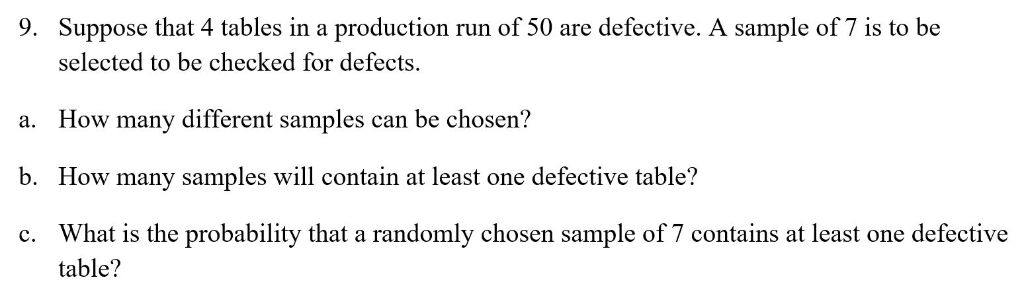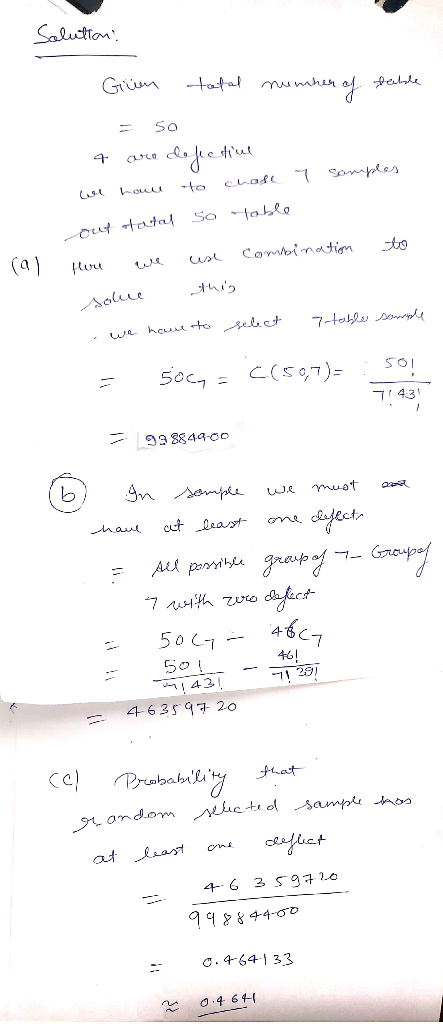# Suppose that 4 tables in a production run of 50 are defective. A sample of 7 is to be selected to be checked for defects 9. How many different samples can be chosen? a. How many samples will contain...Suppose that 4 tables in a production run of 50 are defective. A sample of 7 is to be selected to be checked for defects 9. How many different samples can be chosen? a. How many samples will contain at least one defective table? b. What is the probability that a randomly chosen sample of 7 contains at least one defective table? c.##### Add Answer of: Suppose that 4 tables in a production run of 50 are defective. A sample of 7 is to be selected to be checked for defects 9. How many different samples can be chosen? a. How many samples will contain...
More Homework Help Questions Additional questions in this topic.